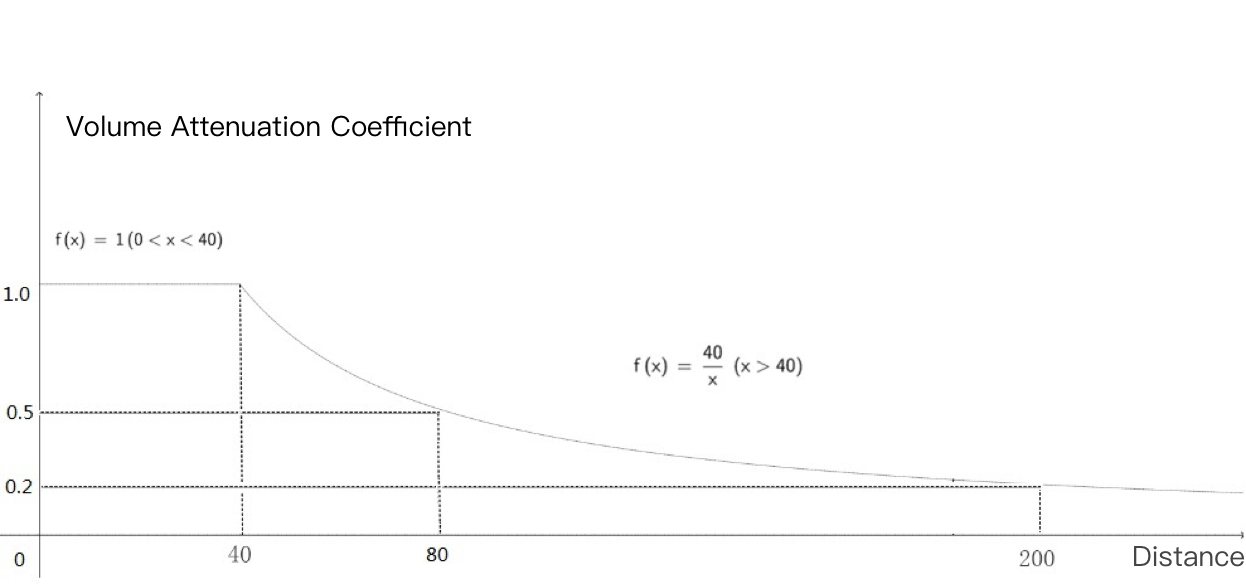# tencent cloud

### 3D Sound Effect

Last updated: 2021-02-26 17:00:23

This document provides a detailed description that makes it easy for developers to debug and integrate GME APIs for 3D sound effect.

## Initialize the 3D sound effect engine

This function is used to initialize the 3D sound effect engine and needs to be called after room entry. You must call this API before using the 3D sound effect. Even if you want to enable only the 3D sound effect reception and not playback, you still need to call this API.

### Function prototype

``public abstract int InitSpatializer(string modelPath)``
Parameter Type Description
modelPath String Path of 3D sound effect resource file

## Enable or disable the 3D sound effect

This function is used to enable or disable the 3D sound effect. You can hear the 3D sound after enabling it.

### Function prototype

``public abstract int EnableSpatializer(bool enable, bool applyToTeam)``
Parameter Type Description
enable Bool You can hear the 3D sound after enabling it.
applyToTeam Bool Indicates whether 3D sound applies to the team. This parameter takes effect only when `enable` is "true".

## Obtain status of 3D sound effect

This function is used to obtain the status of 3D sound effect.

### Function prototype

``public abstract bool IsEnableSpatializer()``
Returned Value Description
true It is enabled.
false It is disabled.

## Update sound source azimuth (including orientation)

This function is used to update the sound source azimuth information. The 3D sound effect can be achieved by calling this function for each frame.

### The relationship between distance and sound attenuation

In the 3D sound effect, the sound will begin to attenuate to almost zero as the distance to the sound source exceeds a specified threshold (range/10).

Distance (Unit in Engine) Attenuation Coefficient
0 < N < range/10 1.0 (no attenuation)
N ≥ range/10 range/10/N### Function prototype

``public abstract void UpdateAudioRecvRange(int range)``
Parameter Type Description
range Int Sets the range within which the sound can be received
``public abstract int UpdateSelfPosition(int position, float axisForward, float axisRight, float axisUp)``
Parameter Type Description
position Int[] The user's X-Y-Z coordinates in the world coordinate system
axisForward Float[] The unit vector of x axis in the coordinate system
axisRight Float[] The unit vector of y axis in the coordinate system
axisUp Float[] The unit vector of z axis in the coordinate system

### Sample code

#### Unreal

``````FVector cameraLocation = UGameplayStatics::GetPlayerCameraManager(GetWorld(), 0)->GetCameraLocation();
FRotator cameraRotation = UGameplayStatics::GetPlayerCameraManager(GetWorld(), 0)->GetCameraRotation();
int position[] = { (int)cameraLocation.X,(int)cameraLocation.Y, (int)cameraLocation.Z };
FMatrix matrix = ((FRotationMatrix)cameraRotation);
float forward[] = { matrix.GetColumn(0).X,matrix.GetColumn(1).X,matrix.GetColumn(2).X };
float right[] = { matrix.GetColumn(0).Y,matrix.GetColumn(1).Y,matrix.GetColumn(2).Y };
float up[] = { matrix.GetColumn(0).Z,matrix.GetColumn(1).Z,matrix.GetColumn(2).Z};

#### Unity

``````Transform selftrans = currentPlayer.gameObject.transform;
Matrix4x4 matrix = Matrix4x4.TRS(Vector3.zero, selftrans.rotation, Vector3.one);
int[] position = new int { selftrans.position.z, selftrans.position.x, selftrans.position.y };
float[] axisForward = new float { matrix.m22, matrix.m02, matrix.m12 };
float[] axisRight = new float { matrix.m20, matrix.m00, matrix.m10 };
float[] axisUp = new float { matrix.m21, matrix.m01, matrix.m11 };